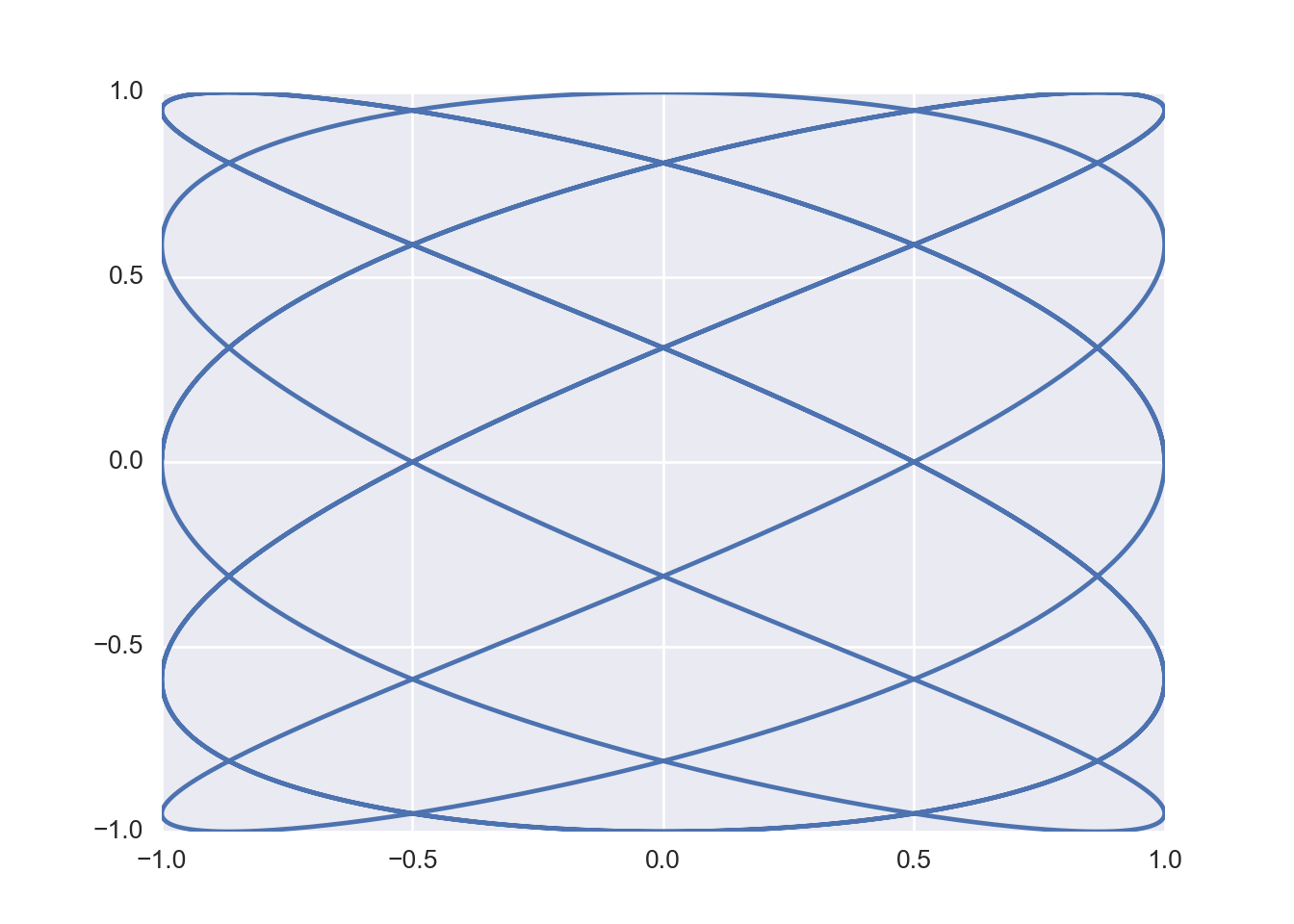## R Markdown

Reticulate is an R library that allows users to embed Python in R. It is very powerful - for example allowing users to include chunks of Python in RMarkdown documents. First off, load the package:

``library(reticulate)``

Then we can start working with Python - (providing it is already there on your system). Here is some Python embedded in the Rmarkdown.

``````import pandas as pd
import numpy as np
import seaborn as sb
meow = np.array([[-1,1,1],[1,1,-1],[1,1,1]])
print(meow)``````
``````## [[-1  1  1]
##  [ 1  1 -1]
##  [ 1  1  1]]``````
``print(meow.shape)``
``## (3, 3)``

You can refrence the python objects you have created using R:

``````library(reticulate)
py\$meow``````
``````##      [,1] [,2] [,3]
## [1,]   -1    1    1
## [2,]    1    1   -1
## [3,]    1    1    1``````
``````woof <- solve(py\$meow)
woof``````
``````##      [,1] [,2] [,3]
## [1,] -0.5  0.0  0.5
## [2,]  0.5  0.5  0.0
## [3,]  0.0 -0.5  0.5``````

The same trick works in the other direction - `woof` is an R object created above…

…. and here it is inside Python:

``print(r.woof)``
``````## [[-0.5  0.   0.5]
##  [ 0.5  0.5  0. ]
##  [ 0.  -0.5  0.5]]``````
``````from numpy.linalg import inv
print(inv(r.woof))``````
``````## [[-1.  1.  1.]
##  [ 1.  1. -1.]
##  [ 1.  1.  1.]]``````
``print(meow - inv(r.woof))``
``````## [[ 0.  0.  0.]
##  [ 0.  0.  0.]
##  [ 0.  0.  0.]]``````

Graphics also works!

``````import matplotlib.pyplot as plt
phi = np.arange(0,3*np.pi,0.0025)
x = np.cos(5*phi)
y = np.sin(3*phi)
plt.plot(x,y)
plt.show()``````Quite fancy stuff…

``````import numpy as np
import matplotlib.pyplot as plt
from matplotlib import cm
from mpl_toolkits.mplot3d import Axes3D
X = np.arange(-5, 5, 0.25)
Y = np.arange(-5, 5, 0.25)
X, Y = np.meshgrid(X, Y)
R = np.sqrt(X**2 + Y**2)
Z = np.sin(R)
fig = plt.figure()
ax = Axes3D(fig)
ax.plot_surface(X, Y, Z, rstride=1, cstride=1, cmap=cm.viridis)
plt.show()``````